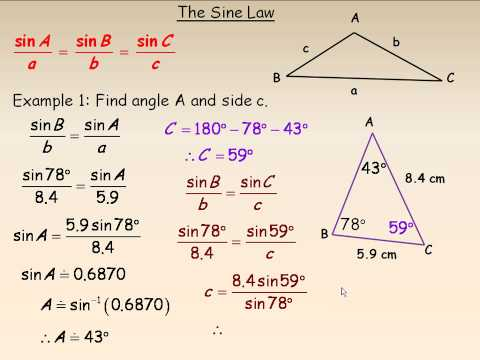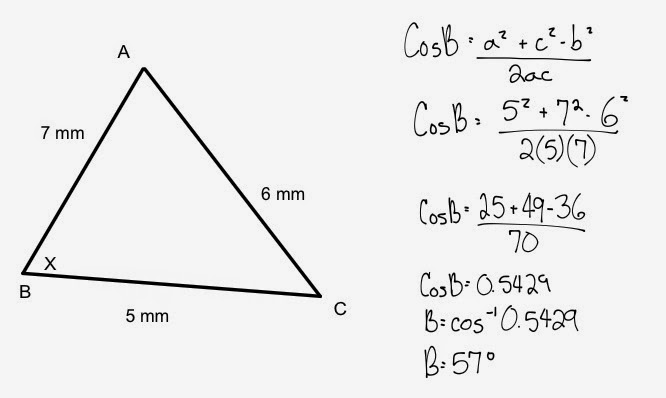# Week 18 – Top 5 things I’ve learned in Pre-Calc 11

1. Finding infinite geometric series values – The most interesting topic that I have learned so far doing math! This shows us the formula to finding the sum of endless series of numbers. It is just mind-blowing how humanity came up with a formula that helps us to find the sum of infinite series of numbers. I just can’t wait to do Pre-Calc 12 and Calc 12, which would have even interesting, and expanded formulas than those in Pre-Calc 11.
2. Analyzing Quadratics – This is a topic where we have to find vertex, the domain, the range, and visualize what the whole graph’s going to turn out to be by solving quadratics. I find this very useful and realized that this will be helpful when improving visualizing skills.
3. Solving Quadratics – This topic expands the factoring skill that we learned during Grade 10, and it is the most useful skills that helped me throughout the semester. Learning how to factor helped me solve problems faster, and easier. It not only helped me in Pre-Calc 11 class but in my Physics class. It also helped me think better and choose wisely as in this topic, there is not only one but many forms of factoring formulas.
4. Solving rational equations – Calculation errors always have been a thing to me whenever it comes to test days. However, this topic taught me again to be careful with subtractions and additions. By far the most complicated topic and only topic that made me anxious on test days due to a possibility of calculation errors.
5. Sine and Cosine law – This topic was new to me and never thought that I would encounter a topic where we dealt with non-right triangles. Dealing with angles, and dimensionally can always be useful in life, and in the future where one dreams of going into space engineering.

# Week 17 – Trigonometry

In this week, we expanded our knowledge of Grade 10 trig. We started off by reviewing Grade 10 trig last week. We went through the formula SOH CAH TOA to remind us about the topic we learned. This week, we learned how to find the length and the angle of a special triangle that isn’t 90 degrees like the ones that we did when we were Grade 10. We learned about the reference angle, the quadrants where the angles located the negative and the positive sides of a triangle , finding the angle and the length with Sine law, and Cosine Law.

Reference angleSine LawCosine Law# Week 16 – Reviewing Trigonometry from Grade 10

This week,  we have went through and reviewed Trigonometry from Grade 10. We learnt that the triangle equals 180 degrees, and the angle changes where the adjacent, and the opposite length will be for that triangle with that angle. This week was a reminder for all of to get us ready for Grade 11 pre-calculus trigonometry where we will be using Sine Law and Cosine Law to find the angle and the lengths of the triangle.

In Grade 10,  we learned the formula SOH CAH TOA

SOH means when there is the opposite length and the hypotenuse, we will be using Sine.

Sine X degree = Opposite/Hypotenuse

CAH means when there is the adjacent length and the hypotenuse, we will be using Cosine.

TOA means when there is the opposite length and the adjacent, we will be using Tangent.

# Week 15 – Solving Rational Equations

This week, we have learned about solving rational equations.

First of all the most important part when solving multiple rational equations is to see whether the denominator’s the same.

For example, lets look at this equation.We can see that to make the equation the same on both sides, (x+6) was multiplied on the left side and (x+3) was multiplied on the right side.

Then, to make sure we have one equation, 8x+24 was moved to the left side and we could see that there is a common of Xs’.

We’ve calculated 6x-8x (-8x is when 8x was moved to the left) and got the result as shown above.

At last, we factored the equation having 2 Xs’

X=6, X=-4

# Week 14 – Rational Expressions and Equations

This week, we learned about rationalizing expressions and equations; linear or quadratic.

We learned that denominator can’t be 0 and because of that there is restrictions, which is also called non-permissible values.

If we look at this expressionWe know that x can’t be either 0 or 5 and when we factor both sides, we can see that there is a common of x-5. So, it gets canceled.

This chapter needs alot of practice with factoring.

# Week 12 – Absolute Value Functions

Absolute Value is a magnitude of real number without regarding to its signs.The absolute value has the form of y =  |f(x)|, where f(x) is a function.

The x-intercept of the graph of y = f(x) is a critical point also known as point of reflection.

So when there is an absolute value symbol, the line always reflects.

As long as we know the concept of an Absolute Value, it is easy to solve problem solving questions.

# Week 11 – Graphing Inequalities

This week I have learned how to solve graphing inequalities; linear and quadratic functions.When the equal sign in a quadratic or linear equation is replaced with an inequality sign, a quadratic inequality in one variable is formed. It also determines the domain and range for one equation. It is a very useful when having graphing skills. I have a example of linear and quadratic equation each above. I find determining whether this equation is true or false hard as I have to plug in random points on the graph, which isn’t labelled, which also determine the shaded parts, depending on whether it is true or false.

# Week 10-Midterm review

This week, it was a review week. We were using our time to answer few questions and ask questions if necessary. While doing reviews, I’ve realized that I forgot about Sequences; Geometric and Arithmetic. The most important ones i realized was that I have to know all the formulas to answer most of the questions. After reviewing through the book, I finally understand the chapter fully.